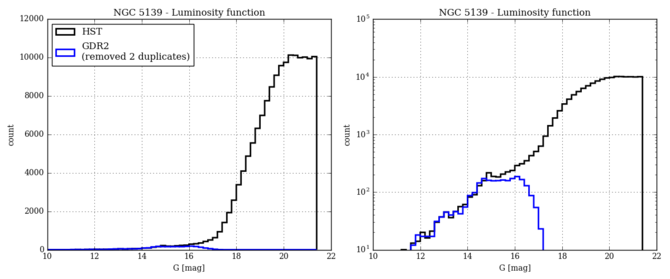# 10.6.4 Completeness

We compare the completeness in different globular clusters with the expected HST completeness, after removing the duplicated sources. No other filtering was applied to the data. The HST magnitudes were converted to the $G$ magnitudes using the relations of Jordi et al. (2010). The zero point of this transformation was adjusted for each cluster using a subset of bright stars in common, in order to avoid variations due to metallicity and interstellar extinction

Figures 10.50, 10.51, and 10.52 present the luminosity function (in HST and Gaia data) for three globulars at different level of crowding. In NGC 6656 the completeness is better than 80% down to $G$ =17 and then drops to 0 at $G$ =18; in NGC 6366 the completeness is around 90% even at 20.5. For NGC 5139 it is about 100% at $G$ =14; 80% near $G$ =15, drops around $G$ =16, down to 0 at $G$ =17.Figure 10.50: Completeness for NGC 6656.Figure 10.51: Same as Figure 10.50, for NGC 5139.Figure 10.52: Same as Figure 10.50, for NGC 6366.

We computed the ratio of number of sources in different magnitude bins, in the inner (inside 0.5 arcmin) and outer (0.5 to 2.2 arcmin) regions of each GC. An example is shown for NGC 6121 (M4) in Figure 10.53. The result for all GCs is presented in Table 10.6. The content of this Table is also presented Figure 10.54, where the double dependence on magnitude and local density can be clearly seen. The completeness of the Gaia DR2 Catalogue is, overall, higher than it was in Gaia DR1.Figure 10.53: Completeness level with respect to HST data in the inner (within 0.5 arcmin) and outer region of the cluster (from Arenou et al. 2018).Figure 10.54: Completeness levels in different regions of 26 globular clusters with different local density, showing the influence of crowding on the completeness (from Arenou et al. 2018).